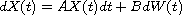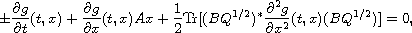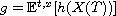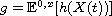Electron. J. Diff. Equ., Vol. 2014 (2014), No. 208, pp. 1-10.

### Feynman-Kac theorem in Hilbert spaces Irina V. Melnikova, Valentina S. Parfenenkova

Abstract:
In this article we study the relationship between solutions to Cauchy problems for the abstract stochastic differential equationand solutions to Cauchy problems (backward and forward) for the infinite dimensional deterministic partial differential equationwhere g is the probability characteristicin the backward case andin the forward case. This relationship, that is the inifinite dimensional Feynman-Kac theorem, is proved in both directions: from stochastic to deterministic and from deterministic to stochastic. Special attention is given to the definition and interpretation of objects in the equations.

Submitted February 20, 2014. Published October 7, 2014.
Math Subject Classifications: 47D06, 60G15, 60H30.
Key Words: Semigroups of operators; infinite dimensional stochastic equations; diffusion processes; Kolmogorov equations.

Show me the PDF file (229 KB), TEX file, and other files for this article.Irina V. Melnikova Ural Federal University Lenin av. 51, 620083 Ekaterinburg, Russia email: Irina.Melnikova@usu.ru Valentina S. Parfenenkova Ural Federal University Lenin av. 51, 620083 Ekaterinburg, Russia email: vika8887@e1.ru